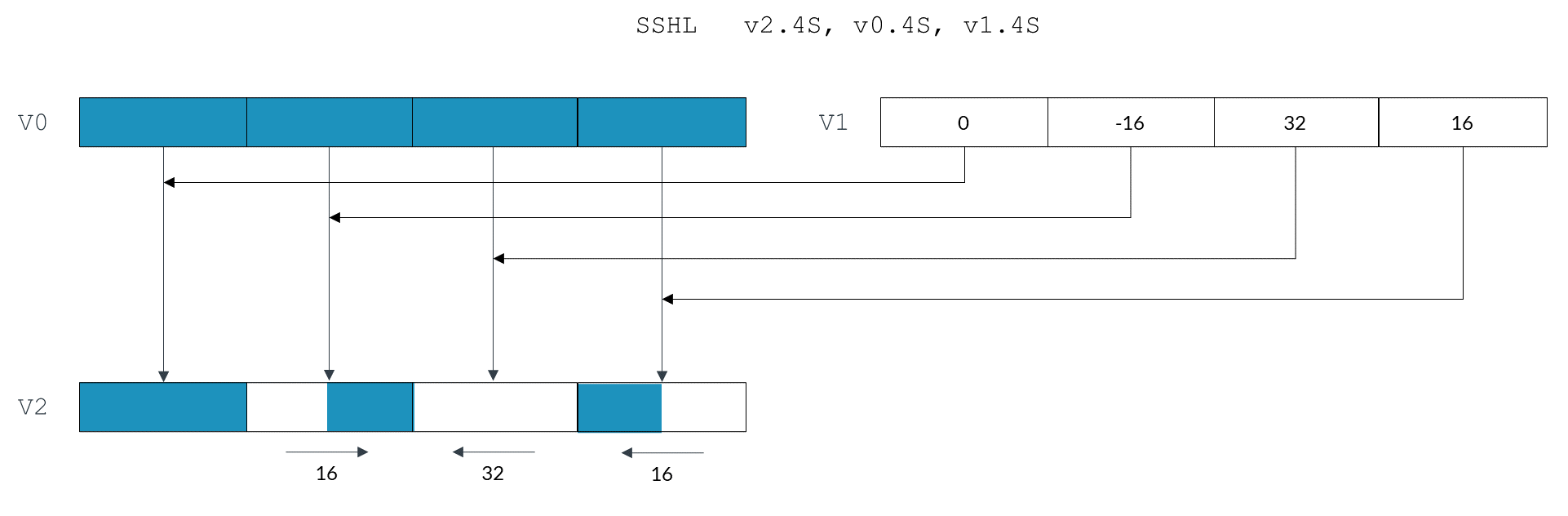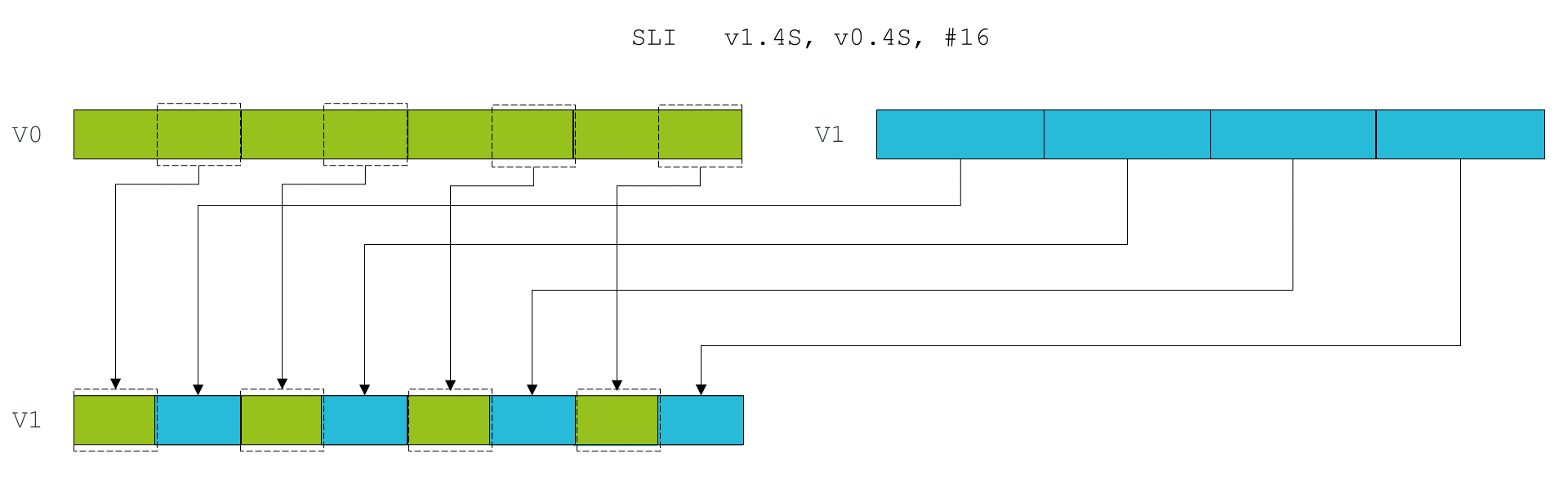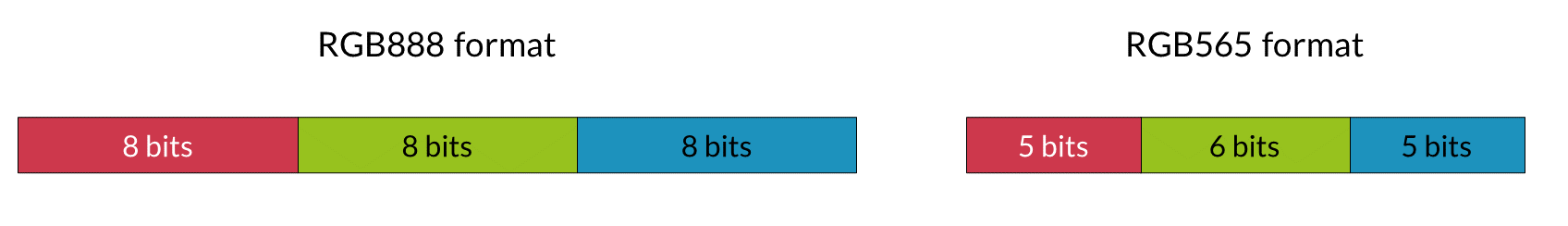## Shifting left and right

This section of the guide introduces the different shift operations that are provided by Neon. An example shows how to use these shifting operations to convert image data between commonly used color depths.

### Shifting vectors

Neon vector shifts are very similar to shifts in scalar Arm code. A shift moves the bits in each element of a vector left or right. Bits that fall off the left or right of each element are discarded. These discarded bits are not shifted to adjacent elements.

The number of bits to shift can be specified as follows:

• With a single immediate literal encoded in the instruction
• With a shift vector

When using a shift vector, the shift that is applied to each element of the input vector depends on the corresponding element in the shift vector. The elements in the shift vector are signed values. This means that left, right, and zero shifts are possible, on a per-element basis. The following diagram shows an input vector, v0, and a shift vector v1:Each vector element shifts as follows:

• Element 0, in the right-most lane of v0, shifts left by 16 bits.
• Element 1 of v0 shifts left by 32 bits. Because the width of the element is also 32 bits, the final value of this element is zero.
• Element 2 of v0 shifts right by 16 bits. The negative value in v1 changes the left shift to a right shift.
• Element 3, in the left-most lane of v0, is unchanged. This is because the zero value in v1 means no shift.

The negative shift value -16 corresponding to element 2 changes the left shift operation to a right shift. When shifting right, we must consider whether we are dealing with signed or unsigned data. Because the `SSHL` instruction is a signed shift operation, the new 16 bits introduced in the top half of this element are the same as the top bit of the original element value. That is, the signed shift `SSHL` is a sign-extending shift. If we use the unsigned `USHL` instruction instead of the signed `SSHL` instruction, the new 16 bits would all be zeroes.

### Shifting and inserting

Neon also supports shifts with insertion. This operation lets you combine bits from two vectors. For example, the `SLI` shift left and insert instruction shifts each element of the source vector left. The new bits that are inserted at the right of each element are the corresponding bits from the destination vector.

The following image shows two vector registers v1 and v2, each containing four elements. The `SLI` instruction takes each element from v1, shifts it left by 16 bits, then combines it with the corresponding element in v0.### Shifting and accumulation

Finally, the Neon instruction `SSRA` supports shifting the elements of a vector right, and accumulating the results into another vector. This instruction is useful for situations in which interim calculations are made at a high precision, before the result is combined with a lower precision accumulator.

### Instruction modifiers

Each shift instruction can take one or more modifiers. These modifiers do not change the shift operation itself, however the inputs or outputs are adjusted to remove bias or saturate to a range.

The general format of shift instructions with modifiers are as follows:

`[<sign>[<sat>]][<round>]SH<dir>[<scale>]`

Where the modifiers are as follows:

Modifier Values Description Example instruction
`<sign>` `S`
`U`

Signed or unsigned.

Specifies whether vector element values are treated as signed or unsigned.

For left shifts, sign does not matter because all bits simply move from right to left. New bits introduced from the right are always zero.

However, negative shift vector values turn a left shift into a right shift. For unsigned data, right shifts use zero for the new bits. For signed data, new bits are the same as the top bit of the original element.

`S` indicates signed. `U` indicates unsigned.

`SSHL` - Signed Shift Left

`USHR` - Unsigned Shift Right
`<sat>` `Q`

Saturating.

Sets each result element to the minimum or maximum of the representable range, if the result exceeds that range. The number of bits and sign type of the vector are used to determine the saturation range.

Unsigned saturating, indicated by a `UQ` prefix, is similar to the saturation modifier. The difference is that the result is saturated to an unsigned range when given signed or unsigned inputs.

`SQSHL` - Signed saturating Shift Left
`<round>` `R`

Rounding.

Specifies whether vector element values are rounded after shifting. This operation corrects for the bias that is caused by truncation when shifting right.

`URSHR` - Unsigned Rounding Shift Right
`<dir>` `L`
`R`
The direction to shift, either left or right. `SHL` - Shift Left

`SRSHR` - Signed Rounding Shift Right
`<scale>` `L`, `L2`
`N`, `N2`

Long (`L`) causes the number of bits in each element of the result to be doubled.

Narrow (`N`) causes the number of bits in each element of the result to be halved.

The suffix modifier `2` indicates an operation on the upper half of either the source register, for narrow instructions, or the destination register, for long instructions.

`SHRN` - Shift Right Narrow

`SHRN2` - Shift Right Narrow (upper)

`SHLL` - Shift Left Long

`SHLL2` - Shift Left Long (upper)

Some combinations of these modifiers do not describe useful operations, so Neon does not provide these instructions. For example, a saturating shift right would be called `UQSHR` or `SQSHR`. However, this operation is unnecessary. Right shifting makes results smaller, so result values can never exceed the available range.

### Available shifting instructions

The following table shows all of the shifting instructions that Neon provides:

Neon instruction Description
`RSHRN`, `RSHRN2` Rounding Shift Right Narrow (immediate).
`SHL` Shift Left (immediate).
`SHLL`, `SHLL2` Shift Left Long (by element size).
`SHRN`, `SHRN2` Shift Right Narrow (immediate).
Shift Left and Insert (immediate).
`SQRSHL` Signed saturating Rounding Shift Left (register).
`SQRSHRN`, `SQRSHRN2` Signed saturating Rounded Shift Right Narrow (immediate).
`SQRSHRUN`, `SQRSHRUN2` Signed saturating Rounded Shift Right Unsigned Narrow (immediate).
`SQSHL` (immediate) Signed saturating Shift Left (immediate).
`SQSHL` (register) Signed saturating Shift Left (register).
`SQSHLU` Signed saturating Shift Left Unsigned (immediate).
`SQSHRN`, `SQSHRN2` Signed saturating Shift Right Narrow (immediate).
`SQSHRUN`, `SQSHRUN2` Signed saturating Shift Right Unsigned Narrow (immediate).
`SRI` Shift Right and Insert (immediate).
`SRSHL` Signed Rounding Shift Left (register).
`SRSHR` Signed Rounding Shift Right (immediate).
`SRSRA` Signed Rounding Shift Right and Accumulate (immediate).
`SSHL` Signed Shift Left (register).
`SSHLL`, `SSHLL2` Signed Shift Left Long (immediate).
`SSHR` Signed Shift Right (immediate).
`SSRA` Signed Shift Right and Accumulate (immediate).
`UQRSHL` Unsigned saturating Rounding Shift Left (register).
`UQRSHRN`, `UQRSHRN2` Unsigned saturating Rounded Shift Right Narrow (immediate).
`UQSHL` (immediate) Unsigned saturating Shift Left (immediate).
`UQSHL` (register) Unsigned saturating Shift Left (register).
`UQSHRN`, `UQSHRN2` Unsigned saturating Shift Right Narrow (immediate).
`URSHL` Unsigned Rounding Shift Left (register).
`URSHR` Unsigned Rounding Shift Right (immediate).
`URSRA` Unsigned Rounding Shift Right and Accumulate (immediate).
`USHL` Unsigned Shift Left (register).
`USHLL`, `USHLL2` Unsigned Shift Left Long (immediate).
`USHR` Unsigned Shift Right (immediate).
`USRA` Unsigned Shift Right and Accumulate (immediate).

### Example: converting color depth

Converting between color depths is a frequent operation in graphics processing. Often, input or output data is in an RGB565 16-bit color format, but working with the data is much easier in RGB888 format. This is particularly true on Neon, because there is no native support for data types like RGB565.

The following diagram shows the RGB888 and RGB565 color formats:However, Neon can still handle RGB565 data efficiently, and the vector shifts introduced in this section provide a method to do this.

#### Converting from RGB565 to RGB888

First, we consider converting RGB565 to RGB888. We assume that there are eight 16-bit pixels in register v0. We want to separate reds, greens, and blues into 8-bit elements across three registers v2 to v4.

The following code uses shift instructions to convert RGB565 to RGB888:

```ushr v1.16b, v0.16b, #3 // Shift red elements right by three bits,
// discarding the green bits at the bottom of
// the red 8-bit elements.

shrn v2.8b, v1.8h, #5   // Shift red elements right and narrow,
// discarding the blue and green bits.

shrn v3.8b, v0.8h, #5   // shift green elements right and narrow
// discarding the blue bits and some red bits
// due to narrowing.

shl v3.8b, v3.8b, #2    // shift green elements left, discarding the
// remaining red bits, and placing green bits
// in the correct place.

shl v0.16b, v0.16b, #3  // shift blue elements left to most significant
// bits of 8-bit color channel.

xtn v4.8b, v0.8h        // remove remaining red and green bits by
// narrowing to 8 bits.```

The effects of each instruction are described in the comments in the preceding code example. In summary, the operation that is performed on each channel is:

1. Remove color data for adjacent channels using shifts to push the bits off either end of the element.
2. Use a second shift to position the color data in the most significant bits of each element.
3. Perform narrowing to reduce the element size from 16-bits to 8-bits.
##### A small problem

You might notice that, if you use this code to convert to RGB888 format, the whites are not quite white. This is because, for each channel, the lowest two or three bits are zero, rather than one. A white represented in RGB565 as (`0x1F`, `0x3F`, `0x1F`) becomes (`0xF8`, `0xFC`, `0xF8`) in RGB888. This can be fixed using shift with insert to place some of the most significant bits into the lower bits.

#### Converting from RGB888 to RGB565

Now, we can look at the reverse operation, converting RGB888 format to RGB565. The RGB888 data is in the format that is produced by the preceding code. Data is separated across three registers v0 to v2, with each vector register containing eight elements of each color. The result is stored as eight 16-bit RGB565 elements in register v3.

The following code converts RGB888 data in registers v0, v1, and v2 to RGB565 data in v3:

```shll v3.8h, v0.8b, #8   // Shift red elements left to most significant
//  bits of wider 16-bit elements.

shll v4.8h, v1.8b, #8   // Shift green elements left to most significant
//  bits of wider 16-bit elements.

sri v3.8h, v4.8h, #5    // Shift green elements right and insert into
//  red elements.

shll v4.8h, v2.8b, #8   // Shift blue elements left to most significant
//  bits of wider 16-bit elements.

sri v3.8h, v4.8h, #11   // Shift blue elements right and insert into
//  red and green elements.```

Again, the detail is in the comments for each instruction in the preceding code, but the process for each channel is as follows:

1. Lengthen each element to 16 bits, and shift the color data into the most significant bits.
2. Use shift right with insert to position each color channel in the result register.

### Conclusion

The powerful range of shift instructions provided by Neon allows you to do the following:

• Quickly divide and multiply vectors by powers of two, with rounding and saturation.
• Shift and copy bits from one vector to another.
• Make interim calculations at high precision and accumulate results at a lower precision.